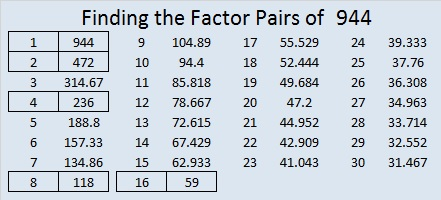# 944 and Level 3

The division facts needed to solve today’s puzzle are not complicated. You can fill in all the cells of this puzzle if you know the multiplication facts from 1 × 1 to 12 × 12.Print the puzzles or type the solution in this excel file: 12 factors 942-950

Now here are some facts about the number 944:

944 is divisible by 2 because it is even.
944 is divisible by 4 because the last number is divisible by 4 and the digit before it is even.
944 can be evenly divided by 8 because 44 is divisible by 4, but not by 8, and the digit before 44 is odd.

944 is a funny-looking palindrome, 1I1, in BASE 23 (I is 18 in base 10) because 1(23²) + 18(23¹) + 1(23⁰) = 944

• 944 is a composite number.
• Prime factorization: 944 = 2 × 2 × 2 × 2 × 59, which can be written 944 = 2⁴ × 59
• The exponents in the prime factorization are 4 and 1. Adding one to each and multiplying we get (4 + 1)(1 + 1) = 5 × 2 = 10. Therefore 944 has exactly 10 factors.
• Factors of 944: 1, 2, 4, 8, 16, 59, 118, 236, 472, 944
• Factor pairs: 944 = 1 × 944, 2 × 472, 4 × 236, 8 × 118, or 16 × 59
• Taking the factor pair with the largest square number factor, we get √944 = (√16)(√59) = 4√59 ≈ 30.72458This site uses Akismet to reduce spam. Learn how your comment data is processed.